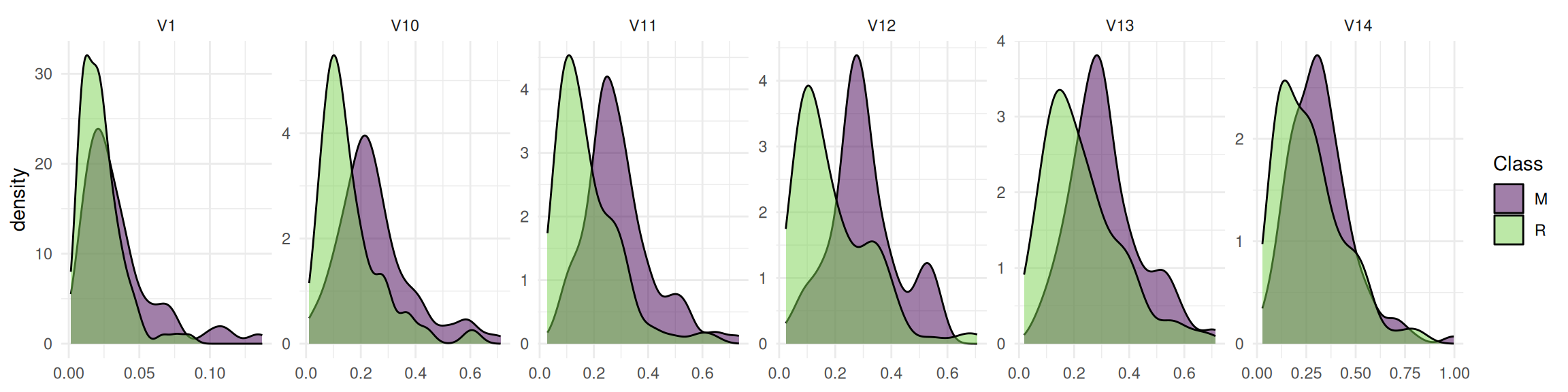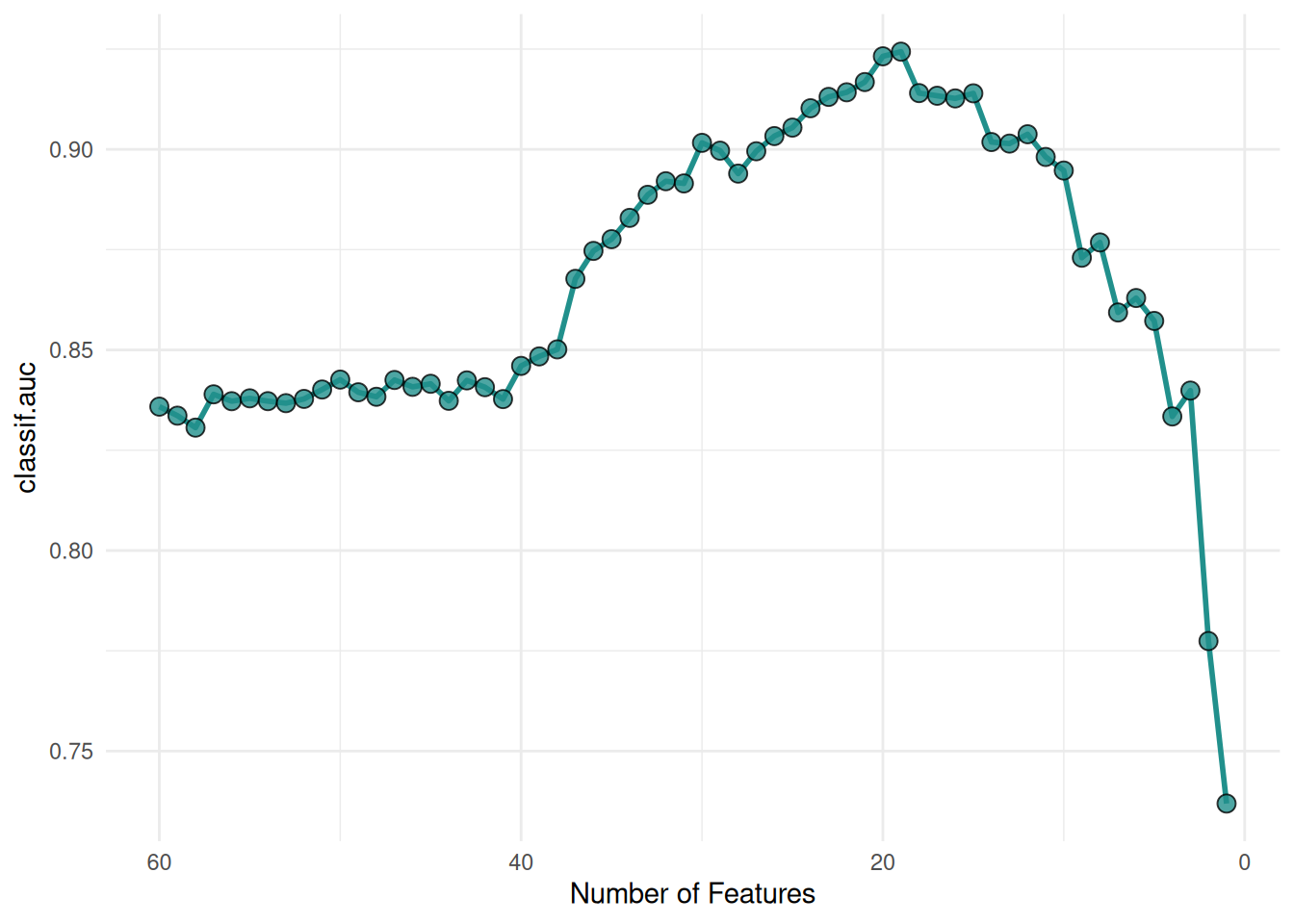# Recursive Feature Elimination on the Sonar Data Set

Utilize the built-in feature importance of models.

Author
Published

February 7, 2023

# Scope

Feature selection is the process of finding an optimal subset of features in order to improve the performance, interpretability and robustness of machine learning algorithms. In this article, we introduce the wrapper feature selection method Recursive Feature Elimination. Wrapper methods iteratively select features that optimize a performance measure. As an example, we will search for the optimal set of features for a `gradient boosting machine` and `support vector machine` on the `Sonar` data set. We assume that you are already familiar with the basic building blocks of the mlr3 ecosystem. If you are new to feature selection, we recommend reading the feature selection chapter of the mlr3book first.

# Recursive Feature Elimination

Recursive Feature Elimination (RFE) is a widely used feature selection method for high-dimensional data sets. The idea is to iteratively remove the least predictive feature from a model until the desired number of features is reached. This feature is determined by the built-in feature importance method of the model. Currently, RFE works with support vector machines (SVM), decision tree algorithms and gradient boosting machines (GBM). Supported learners are tagged with the `"importance"` property. For a full list of supported learners, see the learner page on the mlr-org website and search for `"importance"`.

Guyon et al. (2002) developed the RFE algorithm for SVMs (SVM-RFE) to select informative genes in cancer classification. The importance of the features is given by the weight vector of a linear support vector machine. This method was later extended to other machine learning algorithms. The only requirement is that the models can internally measure the feature importance. The random forest algorithm offers multiple options for measuring feature importance. The commonly used methods are the mean decrease in accuracy (MDA) and the mean decrease in impurity (MDI). The MDA measures the decrease in accuracy for a feature if it was randomly permuted in the out-of-bag sample. The MDI is the total reduction in node impurity when the feature is used for splitting. Gradient boosting algorithms like `XGBoost`, `LightGBM` and `GBM` use similar methods to measure the importance of the features.

Resampling strategies can be combined with the algorithm in different ways. The frameworks scikit-learn and caret implement a variant called recursive feature elimination with cross-validation (RFE-CV) that estimates the optimal number of features with cross-validation first. Then one more RFE is carried out on the complete dataset with the optimal number of features as the final feature set size. The RFE implementation in mlr3 can rank and aggregate importance scores across resampling iterations. We will explore both variants in more detail below.

mlr3fselect is the feature selection package of the mlr3 ecosystem. It implements the `RFE` and `RFE-CV` algorithm. We load all packages of the ecosystem with the `mlr3verse` package.

``library(mlr3verse)``

We retrieve the `RFE` optimizer with the `fs()` function.

``````optimizer = fs("rfe",
n_features = 1,
feature_number = 1,
aggregation = "rank")``````

The algorithm has multiple control parameters. The optimizer stops when the number of features equals `n_features`. The parameters `feature_number`, `feature_fraction` and `subset_size` determine the number of features that are removed in each iteration. The `feature_number` option removes a fixed number of features in each iteration, whereas `feature_fraction` removes a fraction of the features. The `subset_size` argument is a vector that specifies exactly how many features are removed in each iteration. The parameters are mutually exclusive and the default is `feature_fraction = 0.5`. Usually, RFE fits a new model in each resampling iteration and calculates the feature importance again. We can deactivate this behavior by setting `recursive = FALSE`. The selection of feature subsets in all iterations is then based solely on the importance scores of the first model trained with all features. When running an RFE with a resampling strategy like cross-validation, multiple models and importance scores are generated. The `aggregation` parameter determines how the importance scores are aggregated. The option `"rank"` ranks the importance scores in each iteration and then averages the ranks of the features. The feature with the lowest average rank is removed. The option `"mean"` averages the importance scores of the features across the iterations. The `"mean"` should only be used if the learner’s importance scores can be reasonably averaged.

The objective of the `Sonar` data set is to predict whether a sonar signal bounced off a metal cylinder or a rock. The data set includes 60 numerical features (see Figure 1).

``task = tsk("sonar")``
Code
``````library(ggplot2)
library(data.table)

data = data[c("V1", "V10", "V11", "V12", "V13", "V14"), , on = "variable"]

ggplot(data, aes(x = value, fill = Class)) +
geom_density(alpha = 0.5) +
facet_wrap(~ variable, ncol = 6, scales = "free") +
scale_fill_viridis_d(end = 0.8) +
theme_minimal() +
theme(axis.title.x = element_blank())``````Figure 1: Distribution of the first 5 features in the Sonar dataset.

We start with the `GBM learner` and set the predict type to `"prob"` to obtain class probabilities.

``````learner = lrn("classif.gbm",
distribution = "bernoulli",
predict_type = "prob")``````

Now we define the feature selection problem by using the `fsi()` function that constructs an `FSelectInstanceSingleCrit`. In addition to the task and learner, we have to select a `resampling strategy` and `performance measure` to determine how the performance of a feature subset is evaluated. We pass the `"none"` terminator because the `n_features` parameter of the optimizer determines when the feature selection stops.

``````instance = fsi(
learner = learner,
resampling = rsmp("cv", folds = 6),
measures = msr("classif.auc"),
terminator = trm("none"))``````

We are now ready to start the RFE. To do this, we simply pass the instance to the `\$optimize()` method of the optimizer.

``optimizer\$optimize(instance)``

The optimizer saves the best feature set and the corresponding estimated performance in `instance\$result`.

Figure 2 shows the optimization path of the feature selection. We observe that the performance increases first as the number of features decreases. As soon as informative features are removed, the performance drops.

Code
``````library(viridisLite)
library(mlr3misc)

data = as.data.table(instance\$archive)
data[, n:= map_int(importance, length)]

ggplot(data, aes(x = n, y = classif.auc)) +
geom_line(
color = viridis(1, begin = 0.5),
linewidth = 1) +
geom_point(
fill = viridis(1, begin = 0.5),
shape = 21,
size = 3,
stroke = 0.5,
alpha = 0.8) +
xlab("Number of Features") +
scale_x_reverse() +
theme_minimal()``````

The importance scores of the feature sets are recorded in the archive.

``as.data.table(instance\$archive)[, list(features, classif.auc, importance)]``
``````                      features classif.auc                                                importance
1: V1,V10,V11,V12,V13,V14,...   0.8929304 58.83333,58.83333,54.50000,54.00000,53.33333,52.50000,...
2: V1,V10,V11,V12,V13,V15,...   0.9177811 57.33333,56.00000,54.00000,53.66667,50.50000,50.00000,...
3: V1,V10,V11,V12,V13,V15,...   0.9045253 54.83333,54.66667,54.66667,53.00000,51.83333,51.33333,...
4: V1,V10,V11,V12,V13,V15,...   0.8927833 56.00000,55.83333,53.00000,52.00000,50.16667,50.00000,...
5: V1,V10,V11,V12,V13,V15,...   0.9016274 55.50000,53.50000,51.33333,50.00000,49.00000,48.50000,...
---
56:         V11,V12,V16,V48,V9   0.8311625              4.166667,3.333333,2.833333,2.500000,2.166667
57:             V11,V12,V16,V9   0.8216772                       3.833333,2.666667,2.000000,1.500000
58:                V11,V12,V16   0.8065807                                2.833333,1.833333,1.333333
59:                    V11,V12   0.8023780                                         1.833333,1.166667
60:                        V11   0.7515904                                                         1``````

# Support Vector Machine

Now we will select the optimal feature set for an SVM with a linear kernel. The importance scores are the weights of the model.

``````learner = lrn("classif.svm",
type = "C-classification",
kernel = "linear",
predict_type = "prob")``````

The `SVM learner` does not support the calculation of importance scores at first. The reason is that importance scores cannot be determined with all kernels. This can be seen by the missing `"importance"` property.

``learner\$properties``
`` "multiclass" "twoclass"  ``

Using the `"mlr3fselect.svm_rfe"` callback however makes it possible to use a linear SVM with the `RFE` optimizer. The callback adds the `\$importance()` method internally to the learner. We load the callback with the `clbk()` function and pass it as the `"callback"` argument to `fsi()`.

``````instance = fsi(
learner = learner,
resampling = rsmp("cv", folds = 6),
measures = msr("classif.auc"),
terminator = trm("none"),
callback = clbk("mlr3fselect.svm_rfe"))``````

We start the feature selection.

``optimizer\$optimize(instance)``

Figure 3 shows the average performance of the SVMs depending on the number of features. We can see that the performance increases significantly with a reduced feature set.

Code
``````library(viridisLite)
library(mlr3misc)

data = as.data.table(instance\$archive)
data[, n:= map_int(importance, length)]

ggplot(data, aes(x = n, y = classif.auc)) +
geom_line(
color = viridis(1, begin = 0.5),
linewidth = 1) +
geom_point(
fill = viridis(1, begin = 0.5),
shape = 21,
size = 3,
stroke = 0.5,
alpha = 0.8) +
xlab("Number of Features") +
scale_x_reverse() +
theme_minimal()``````Figure 3: Performance of the support vector machines depending on the number of features.

For datasets with a lot of features, it is more efficient to remove several features per iteration. We show an example where 25% of the features are removed in each iteration.

``````optimizer = fs("rfe", n_features = 1, feature_fraction = 0.75)

instance = fsi(
learner = learner,
resampling = rsmp("cv", folds = 6),
measures = msr("classif.auc"),
terminator = trm("none"),
callback = clbk("mlr3fselect.svm_rfe"))

optimizer\$optimize(instance)``````

Figure 4 shows a similar optimization curve as Figure 3 but with fewer evaluated feature sets.

Code
``````library(viridisLite)
library(mlr3misc)

data = as.data.table(instance\$archive)
data[, n:= map_int(importance, length)]

ggplot(data, aes(x = n, y = classif.auc)) +
geom_line(
color = viridis(1, begin = 0.5),
linewidth = 1) +
geom_point(
fill = viridis(1, begin = 0.5),
shape = 21,
size = 3,
stroke = 0.5,
alpha = 0.8) +
xlab("Number of Features") +
scale_x_reverse() +
theme_minimal()``````

# Recursive Feature Elimination with Cross Validation

RFE-CV estimates the optimal number of features before selecting a feature set. For this, an RFE is run in each resampling iteration and the number of features with the best mean performance is selected (see Figure 5). Then one more RFE is carried out on the complete dataset with the optimal number of features as the final feature set size.

We retrieve the `RFE-CV` optimizer. RFE-CV has almost the same control parameters as the RFE optimizer. The only difference is that no aggregation is needed.

``````optimizer = fs("rfecv",
n_features = 1,
feature_number = 1)``````

The chosen resampling strategy is used to estimate the optimal number of features. The 6-fold cross-validation results in 6 RFE runs. You can choose any other resampling strategy with multiple iterations. Let’s start the feature selection.

``````learner = lrn("classif.svm",
type = "C-classification",
kernel = "linear",
predict_type = "prob")

instance = fsi(
learner = learner,
resampling = rsmp("cv", folds = 6),
measures = msr("classif.auc"),
terminator = trm("none"),
callback = clbk("mlr3fselect.svm_rfe"))

optimizer\$optimize(instance)``````
Warning

The performance of the optimal feature set is calculated on the complete data set and should not be reported as the performance of the final model. Estimate the performance of the final model with nested resampling.

We visualize the selection of the optimal number of features. Each point is the mean performance of the number of features. We achieved the best performance with 19 features.

Code
``````library(ggplot2)
library(viridisLite)
library(mlr3misc)

data = as.data.table(instance\$archive)[!is.na(iteration), ]
aggr = data[, list("y" = mean(unlist(.SD))), by = "batch_nr", .SDcols = "classif.auc"]
aggr[, batch_nr := 61 - batch_nr]

data[, n:= map_int(importance, length)]

ggplot(aggr, aes(x = batch_nr, y = y)) +
geom_line(
color = viridis(1, begin = 0.5),
linewidth = 1) +
geom_point(
fill = viridis(1, begin = 0.5),
shape = 21,
size = 3,
stroke = 0.5,
alpha = 0.8) +
geom_vline(
xintercept = aggr[y == max(y)]\$batch_nr,
colour = viridis(1, begin = 0.33),
linetype = 3
) +
xlab("Number of Features") +
ylab("Mean AUC") +
scale_x_reverse() +
theme_minimal()``````Figure 6: Estimation of the optimal number of features. The best mean performance is achieved with 19 features (blue line).

The archive contains the extra column `"iteration"` that indicates in which resampling iteration the feature set was evaluated. The feature subsets of the final RFE run have no value in the `"iteration"` column because they were evaluated on the complete data set.

``as.data.table(instance\$archive)[, list(features, classif.auc, iteration, importance)]``
``````                       features classif.auc iteration                                                      importance
1: V1,V10,V11,V12,V13,V14,...   0.8782895         1       2.864018,1.532774,1.408485,1.399930,1.326165,1.167745,...
2: V1,V10,V11,V12,V13,V14,...   0.7026144         2       2.056442,1.706077,1.258703,1.191762,1.190752,1.178514,...
3: V1,V10,V11,V12,V13,V14,...   0.8790850         3       1.950412,1.887710,1.820891,1.616219,1.231928,1.138675,...
4: V1,V10,V11,V12,V13,V14,...   0.8125000         4 2.6958580,1.5623759,1.4990138,1.3902109,0.9385757,0.9232132,...
5: V1,V10,V11,V12,V13,V14,...   0.8807018         5       2.487483,1.470778,1.356517,1.033764,0.635383,0.575074,...
---
398:  V1,V11,V12,V16,V23,V3,...   0.9605275        NA 2.0089739,1.1047492,1.0011253,0.6602411,0.6015470,0.5431803,...
399:  V1,V12,V16,V23,V3,V30,...   0.9595988        NA 1.8337471,1.1937962,0.9853467,0.7751384,0.7296726,0.6222569,...
400:  V1,V12,V16,V23,V3,V30,...   0.9589486        NA 1.8824952,1.2468164,1.0106654,0.8090618,0.6983925,0.6568389,...
401:  V1,V12,V16,V23,V3,V30,...   0.9559766        NA 2.3872902,0.9094028,0.8809098,0.8277941,0.7841591,0.7792772,...
402:  V1,V12,V16,V23,V3,V30,...   0.9521687        NA 1.9485133,1.1482257,1.1098823,0.9591012,0.8234140,0.8118616,...``````

# Final Model

The learner we use to make predictions on new data is called the final model. The final model is trained with the optimal feature set on the full data set. The optimal set consists of 19 features and is stored in `instance\$result_feature_set`. We subset the task to the optimal feature set and train the learner.

``````task\$select(instance\$result_feature_set)